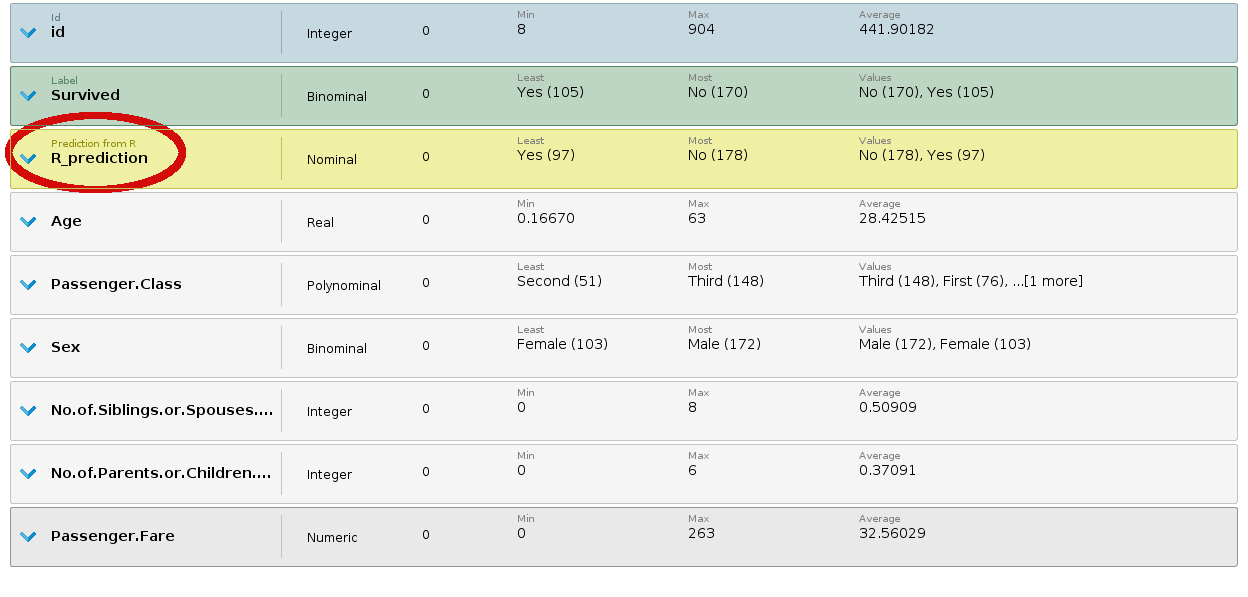Using the special roles of the ExampleSet inside an R script

Administrator, Moderator, Employee, RMResearcher, Member Posts: 277RM Research
edited December 2018

Embedding your R scripts into RapidMiner is a great way to provide even more data science and machine learning assets for your usage.

The other interesting fact is, that by using the RapidMiner ecosystem you can easily operationalize your scripts. Because embedding your scripts into a production ready system can be harder than imagined. To cope with this task, RapidMiner offers some advanced features when processing R scripts. For example meta data about the role of an Attribute.

Using Roles

Special roles in an ExampleSet can help to organize the data and clarify the data mining work flow. For example data sets often contain an ID attribute. An ID is useful for backtracking the data to its source. On the other hand including the ID for training a classifier is a common mistake, that all data scientist have to be done at least once.

RapidMiner helps here by handling roles and special attribute types. Those can be set by the user via the “Set Role” Operator, for example to declare an attribute as label or as ID. Also the results of machine learning Operators normally have a new attribute “Prediction”.

When passing an ExampleSet to the “Execute R" Operator, those information are preserved. They can also be edited, so the roles of the output data matches those of an actual RapidMiner Operator. For example, we can set the role of a newly generated attribute. One use case is to set the output of a learner to “Prediction”, so then you can directly calculate a performance measure in RapidMiner.

The following Process shows how you can you use the rpart library of R to classify the Iris data set:

Spoiler

<?xml version="1.0" encoding="UTF-8"?><process version="8.1.000">
<context>
<input/>
<output/>
<macros/>
</context>
<operator activated="true" class="process" compatibility="8.1.000" expanded="true" name="Process">
<process expanded="true">
<operator activated="true" class="retrieve" compatibility="8.1.000" expanded="true" height="68" name="Retrieve Iris" width="90" x="112" y="34">
<parameter key="repository_entry" value="//Samples/data/Iris"/>
</operator>

<operator activated="true" class="split_data" compatibility="8.1.000" expanded="true" height="103" name="Split Data" width="90" x="246" y="34">
<enumeration key="partitions">
<parameter key="ratio" value="0.7"/>
<parameter key="ratio" value="0.3"/>
</enumeration>
<parameter key="sampling_type" value="stratified sampling"/>
<description align="center" color="transparent" colored="false" width="126">Split the data into training and test sets</description>
</operator>

<operator activated="true" class="r_scripting:execute_r" compatibility="8.1.000" expanded="true" height="103" name="Execute R" width="90" x="581" y="34">
<parameter key="script" value="library(&quot;rpart&quot;)&#10;&#10;rm_main = function(training, test) {&#10;&#10;&#9;## identify the label attribute by its meta data and extract the name as a variable&#10;&#9;labelEntry &lt;- grep(&quot;label&quot;,metaData\$training)&#10;&#9;labelName &lt;- names(metaData\$training[labelEntry])&#10;&#10;&#9;## identify the ID attribute by its meta data and extract the name as a variable&#10;&#9;idEntry &lt;- grep(&quot;id&quot;,metaData\$training)&#10;&#9;idName &lt;- names(metaData\$training[idEntry])&#10;&#10;&#10;&#9;## use the labelName as target variable for a model formula&#10; formula &lt;- as.formula(paste0(labelName, &quot;~ .&quot;))&#10;&#10;&#9;## build the tree model on the test data&#10;&#9;## exclude the id Attribute from the data&#10; treeModel &lt;- rpart(formula, data=subset(training, select = -get(idName)), method=&quot;class&quot;)&#10;&#10; ## apply the model on the test data&#10;&#9;prediction &lt;- as.vector(predict(treeModel, newdata=subset(test, select = -get(idName)), type=&quot;class&quot;))&#10;&#10;&#9;## add the prediction to the test data&#10;&#9;test\$prediction &lt;- prediction&#10;&#10; &#9;# update the meta data&#10;&#9;metaData\$test\$prediction &lt;&lt;- list(type=&quot;nominal&quot;, role=&quot;prediction&quot;)&#10;&#9;&#10; &#9;return(list(test=test))&#10; &#10;}&#10;"/>
</operator>

<operator activated="true" class="performance_classification" compatibility="8.1.000" expanded="true" height="82" name="Performance " width="90" x="782" y="34">
<list key="class_weights"/>
</operator>

<connect from_op="Retrieve Iris" from_port="output" to_op="Split Data" to_port="example set"/>
<connect from_op="Split Data" from_port="partition 1" to_op="Execute R" to_port="input 1"/>
<connect from_op="Split Data" from_port="partition 2" to_op="Execute R" to_port="input 2"/>
<connect from_op="Execute R" from_port="output 1" to_op="Performance (2)" to_port="labelled data"/>
<connect from_op="Performance (2)" from_port="performance" to_port="result 1"/>
<portSpacing port="source_input 1" spacing="0"/>
<portSpacing port="sink_result 1" spacing="0"/>
<portSpacing port="sink_result 2" spacing="0"/>
<description align="center" color="green" colored="true" height="211" resized="true" width="383" x="455" y="151">Look at the parameter 'script' to see how to handle meta data.&lt;br&gt;The idea is to build a simple classification model on the training data and apply it on the test data.&lt;br&gt;&lt;br&gt;The meta data are used to:&lt;br/&gt;&lt;br&gt;i) identify label attribute for the learning task and also to exclude the ID from the model.&lt;br&gt;&lt;br&gt;ii) set the role of the classifier output to &amp;quot;prediction&amp;quot;</description>
</process>
</operator>
</process>

And this is the used R script:

Spoiler

library("rpart")

rm_main = function(training, test) {

## identify the label attribute by its meta data and extract the name as a variable

## identify the ID attribute by its meta data and extract the name as a variable

## use the labelName as target variable for a model formula
formula <- as.formula(paste0(labelName, "~ ."))

## build the tree model on the test data
## and exclude the id Attribute from the data
treeModel <- rpart(formula, data=subset(training, select = -get(idName)), method="class")

## apply the model on the test data
prediction <- as.vector(predict(treeModel, newdata=subset(test, select = -get(idName)), type="class"))

## add the prediction to the test data
test\$prediction <- prediction

# update the meta data

return(list(test=test))
}

To access or change a specific meta data entry use

or

, this can be used to either change the meta data for existing entries or add new entries

Please note that changes to the meta data have to be made with the 'superassignment' operator <<- because the meta data lies in a higher namespace.

+Output of the R script, with a new role

Tagged: